# RS Aggarwal Solutions for Class 6 Maths Chapter 2 Factors and Multiples Exercise 2F

RS Aggarwal Solutions for Class 6 Maths Chapter 2 Factors and Multiples Exercise 2F are available in simple PDF for students to refer. The solutions are based on the current CBSE syllabus, which helps in the exam preparation. It mainly helps students grasp better knowledge about the concepts which are covered here. By practicing RS Aggarwal Solutions, students can improve their self-study methods. RS Aggarwal Solutions for Class 6 Chapter 2 Factors and Multiples Exercise 2F can be downloaded by the students in simple PDF format.

## Download PDF of RS Aggarwal Solutions for Class 6 Maths Chapter 2 Factors and Multiples Exercise 2F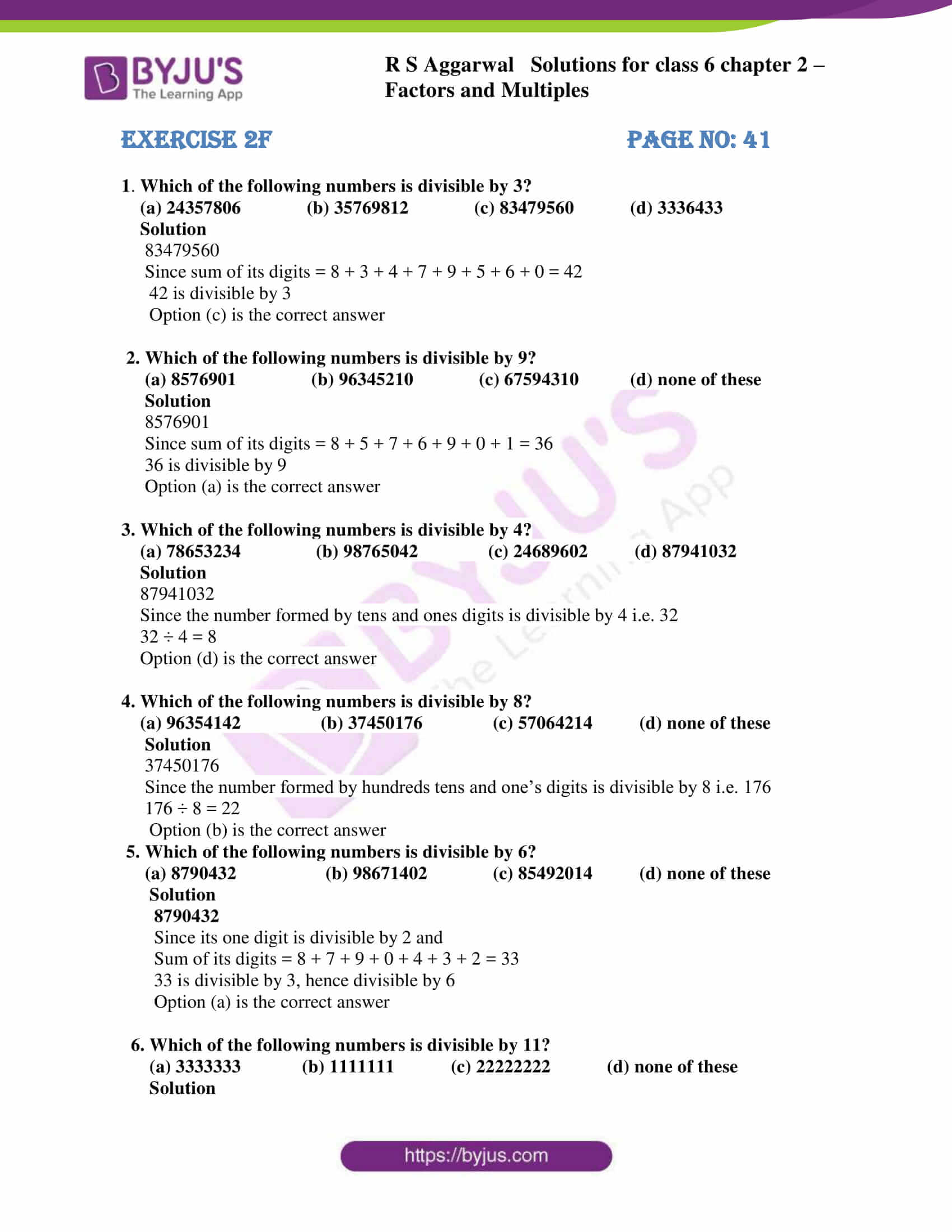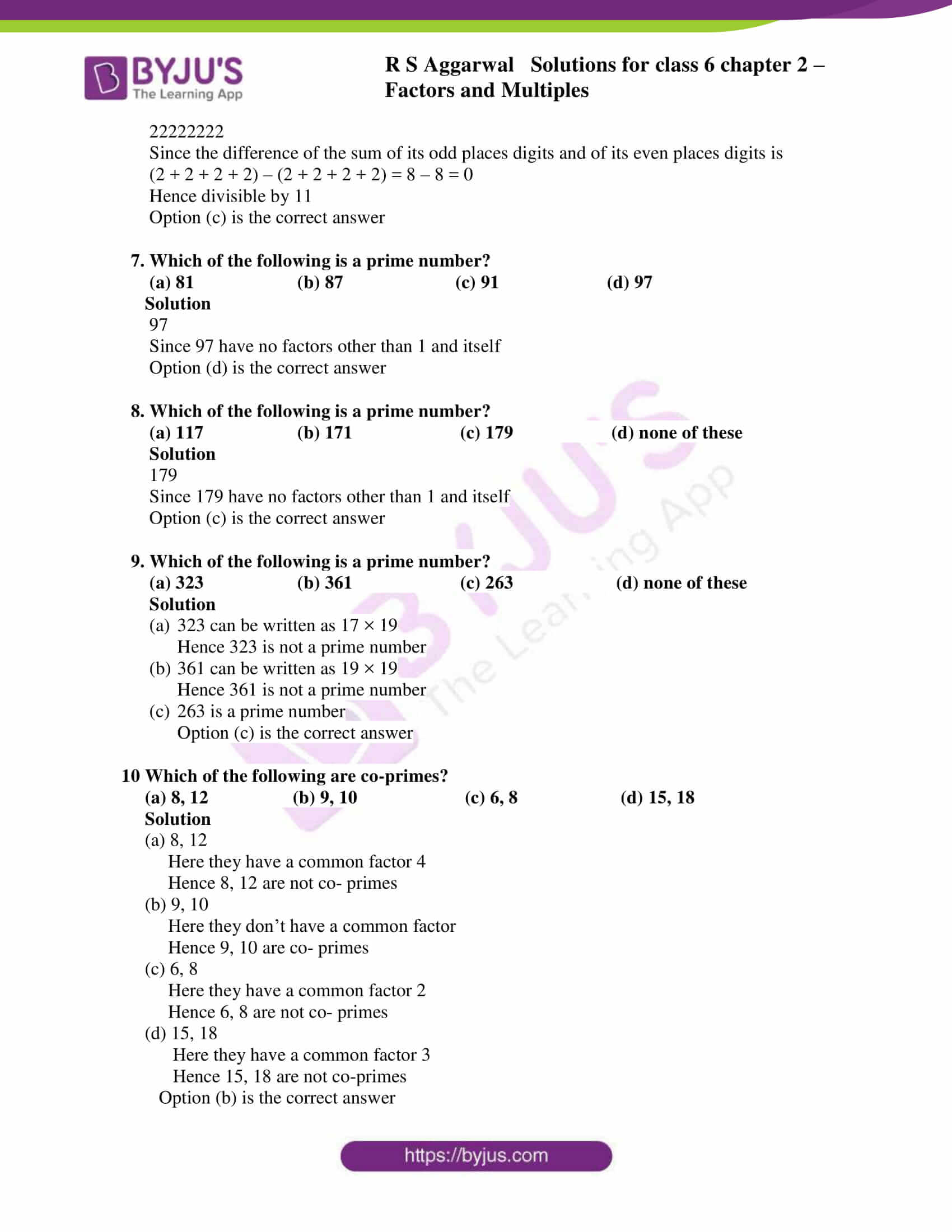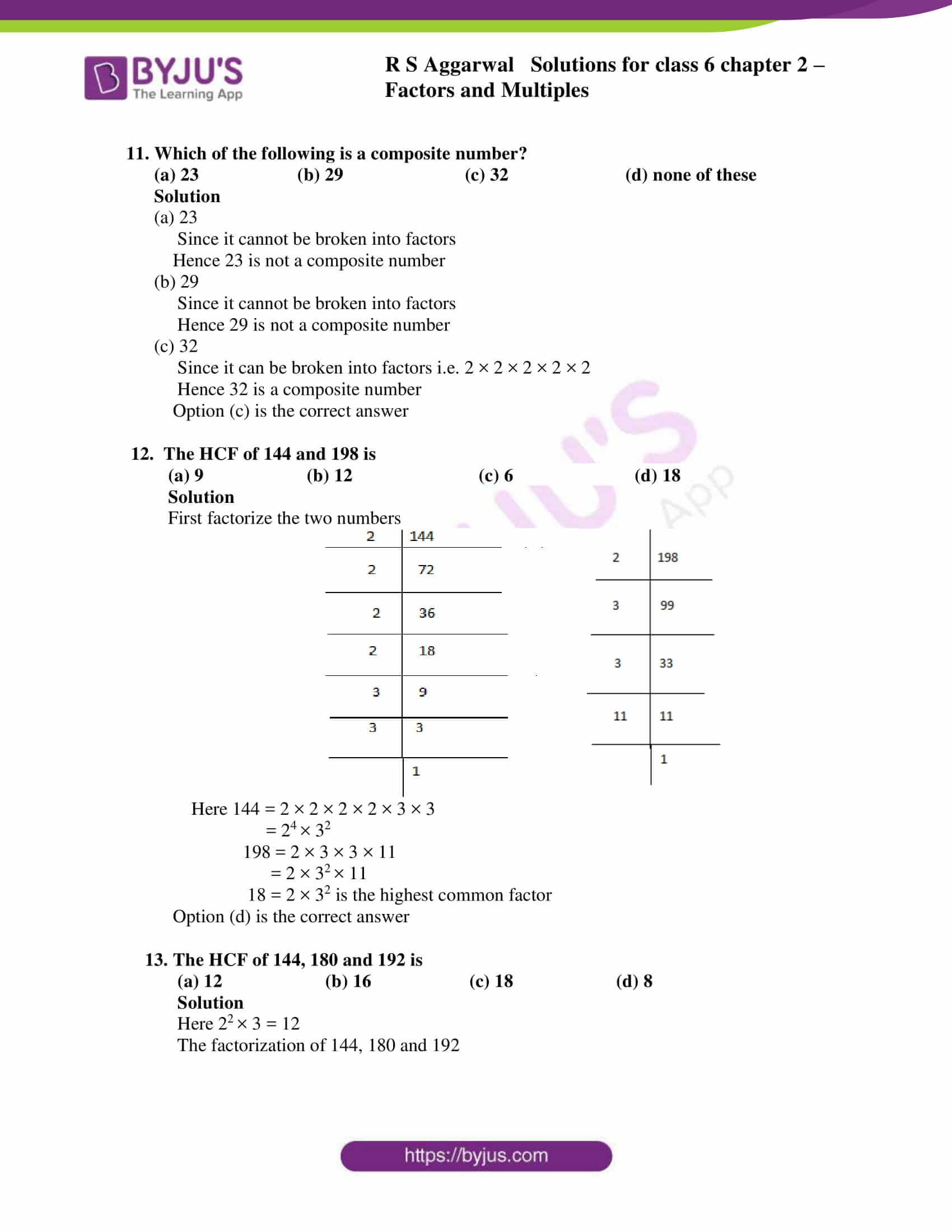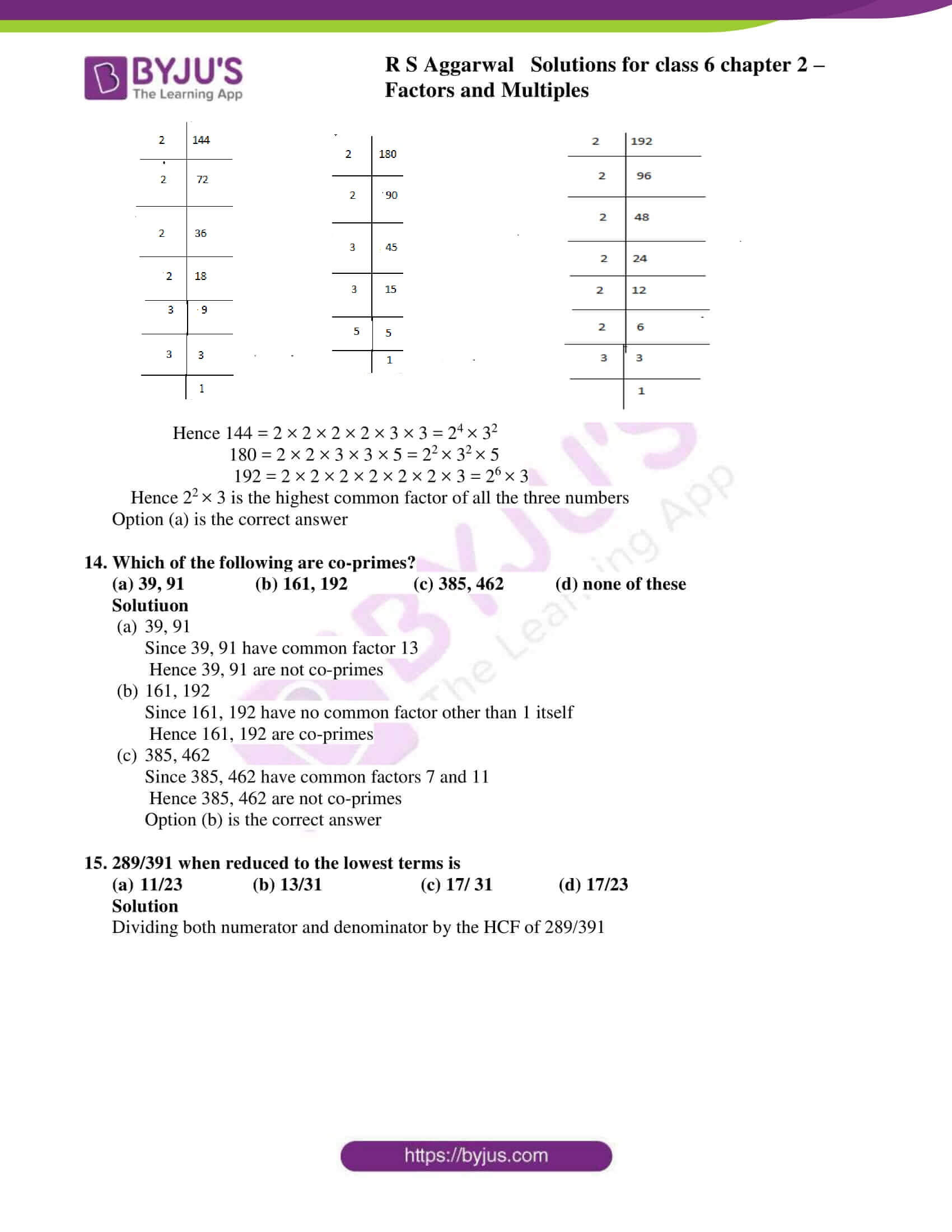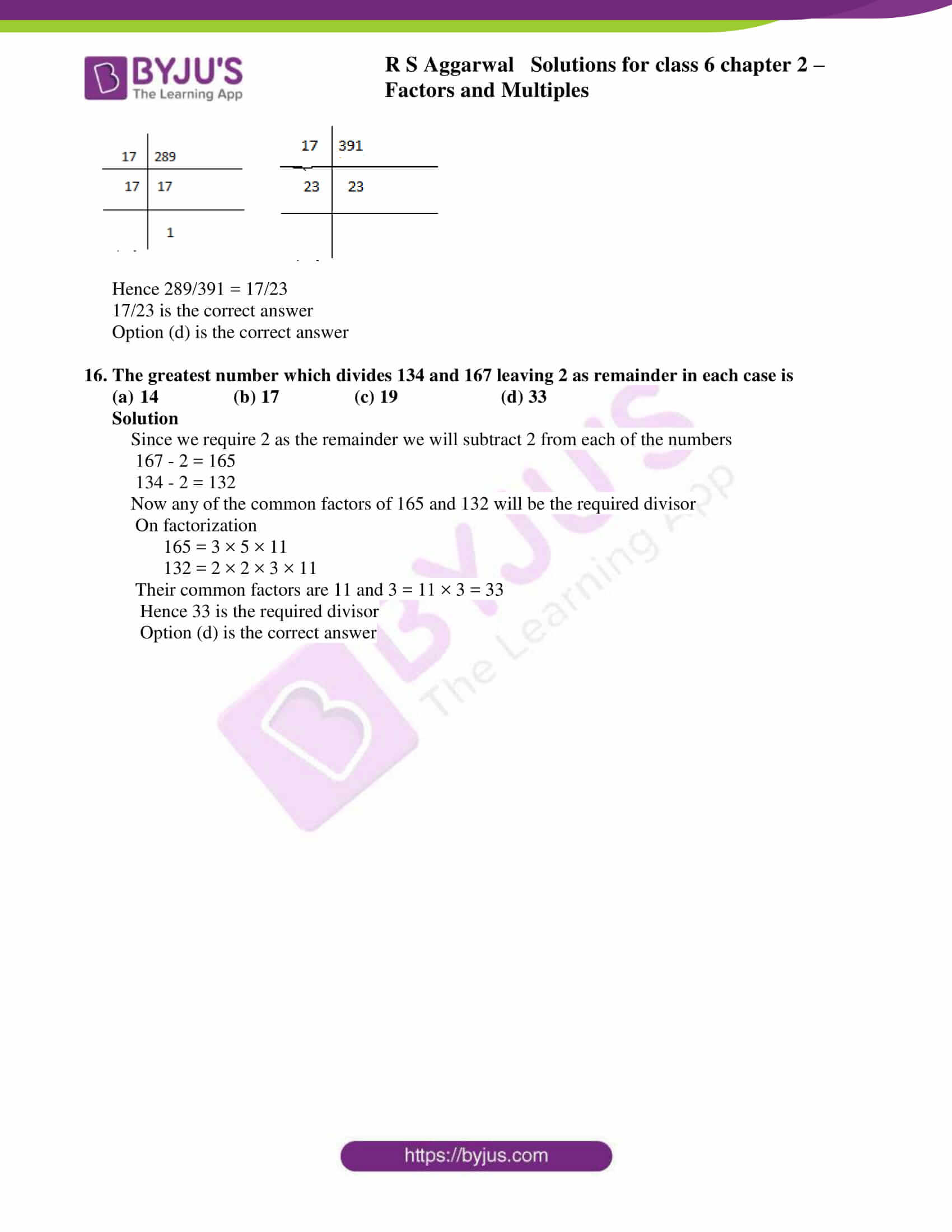### Access answers to Maths RS Aggarwal Solutions for Class 6 Chapter 2 Factors and Multiples Exercise 2F

1. Which of the following numbers is divisible by 3?

(a) 24357806 (b) 35769812 (c) 83479560 (d) 3336433

Solution

83479560

Since sum of its digits = 8 + 3 + 4 + 7 + 9 + 5 + 6 + 0 = 42

42 is divisible by 3

Option (c) is the correct answer

2. Which of the following numbers is divisible by 9?

(a) 8576901 (b) 96345210 (c) 67594310 (d) none of these

Solution

8576901

Since sum of its digits = 8 + 5 + 7 + 6 + 9 + 0 + 1 = 36

36 is divisible by 9

Option (a) is the correct answer

3. Which of the following numbers is divisible by 4?

(a) 78653234 (b) 98765042 (c) 24689602 (d) 87941032

Solution

87941032

Since the number formed by tens and ones digits is divisible by 4 i.e. 32

32 ÷ 4 = 8

Option (d) is the correct answer

4. Which of the following numbers is divisible by 8?

(a) 96354142 (b) 37450176 (c) 57064214 (d) none of these

Solution

37450176

Since the number formed by hundreds tens and one’s digits is divisible by 8 i.e. 176

176 ÷ 8 = 22

Option (b) is the correct answer

5. Which of the following numbers is divisible by 6?

(a) 8790432 (b) 98671402 (c) 85492014 (d) none of these

Solution

8790432

Since its one digit is divisible by 2 and

Sum of its digits = 8 + 7 + 9 + 0 + 4 + 3 + 2 = 33

33 is divisible by 3, hence divisible by 6

Option (a) is the correct answer

6. Which of the following numbers is divisible by 11?

(a) 3333333 (b) 1111111 (c) 22222222 (d) none of these

Solution

22222222

Since the difference of the sum of its odd places digits and of its even places digits is

(2 + 2 + 2 + 2) – (2 + 2 + 2 + 2) = 8 – 8 = 0

Hence divisible by 11

Option (c) is the correct answer

7. Which of the following is a prime number?

(a) 81 (b) 87 (c) 91 (d) 97

Solution

c) 97

Since 97 have no factors other than 1 and itself

Option (d) is the correct answer

8. Which of the following is a prime number?

(a) 117 (b) 171 (c) 179 (d) none of these

Solution

179

Since 179 have no factors other than 1 and itself

Option (c) is the correct answer

9. Which of the following is a prime number?

(a) 323 (b) 361 (c) 263 (d) none of these

Solution

a) 323 can be written as 17 × 19

Hence 323 is not a prime number

b) 361 can be written as 19 × 19

Hence 361 is not a prime number

c) 263 is a prime number

Option (c) is the correct answer

10 Which of the following are co-primes?

(a) 8, 12 (b) 9, 10 (c) 6, 8 (d) 15, 18

Solution

(a) 8, 12

Here they have a common factor 4

Hence 8, 12 are not co- primes

(b) 9, 10

Here they don’t have a common factor

Hence 9, 10 are co- primes

(c) 6, 8

Here they have a common factor 2

Hence 6, 8 are not co- primes

(d) 15, 18

Here they have a common factor 3

Hence 15, 18 are not co-primes

Option (b) is the correct answer

11. Which of the following is a composite number?

(a) 23 (b) 29 (c) 32 (d) none of these

Solution

(a) 23

Since it cannot be broken into factors

Hence 23 is not a composite number

(b) 29

Since it cannot be broken into factors

Hence 29 is not a composite number

(c) 32

Since it can be broken into factors i.e. 2 × 2 × 2 × 2 × 2

Hence 32 is a composite number

Option (c) is the correct answer

12. The HCF of 144 and 198 is

(a) 9 (b) 12 (c) 6 (d) 18

Solution

First factorize the two numbers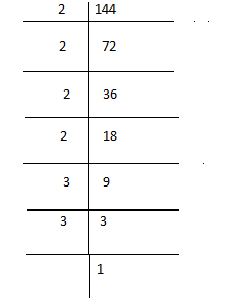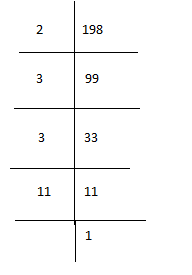Here 144 = 2 × 2 × 2 × 2 × 3 × 3

= 24 × 32

198 = 2 × 3 × 3 × 11

= 2 × 32 × 11

18 = 2 × 32 is the highest common factor

Option (d) is the correct answer

13. The HCF of 144, 180 and 192 is

(a) 12 (b) 16 (c) 18 (d) 8

Solution

Here 22 × 3 = 12

The factorization of 144, 180 and 192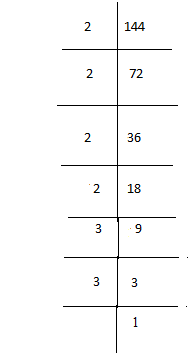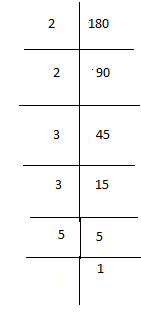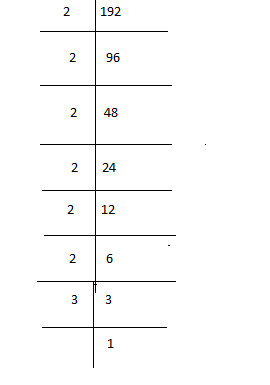Hence 144 = 2 × 2 × 2 × 2 × 3 × 3 = 24 × 32

180 = 2 × 2 × 3 × 3 × 5 = 22 × 32 × 5

192 = 2 × 2 × 2 × 2 × 2 × 2 × 3 = 26 × 3

Hence 22 × 3 is the highest common factor of all the three numbers

Option (a) is the correct answer

14. Which of the following are co-primes?

(a) 39, 91 (b) 161, 192 (c) 385, 462 (d) none of these

Solutiuon

a) 39, 91

Since 39, 91 have common factor 13

Hence 39, 91 are not co-primes

b) 161, 192

Since 161, 192 have no common factor other than 1 itself

Hence 161, 192 are co-primes

c) 385, 462

Since 385, 462 have common factors 7 and 11

Hence 385, 462 are not co-primes

Option (b) is the correct answer

15. 289/391 when reduced to the lowest terms is

(A)11/23 (b) 13/31 (c) 17/ 31 (d) 17/23

Solution

Dividing both numerator and denominator by the HCF of 289/391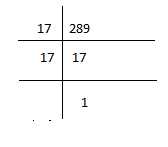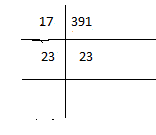Hence 289/391 = 17/23

Option (d) is the correct answer

16. The greatest number which divides 134 and 167 leaving 2 as remainder in each case is

(A)14 (b) 17 (c) 19 (d) 33

Solution

Since we require 2 as the remainder we will subtract 2 from each of the numbers

167 – 2 = 165

134 – 2 = 132

Now any of the common factors of 165 and 132 will be the required divisor

On factorization

165 = 3 × 5 × 11

132 = 2 × 2 × 3 × 11

Their common factors are 11 and 3 = 11 × 3 = 33

Hence 33 is the required divisor

Option (d) is the correct answer

## RS Aggarwal Solutions for Class 6 Maths Chapter 2 Factors and Multiples Exercise 2F

RS Aggarwal Solutions for Class 6 Maths Chapter 2 Factors and Multiples Exercise 2F consists of objective type questions which helps students self analyse their performance. Topics in other exercises are as follows

• Finding prime and composite numbers
• General properties of divisibility
• Prime factorization
• To find HCF
• Properties of HCF and LCM of given numbers.

RS Aggarwal Solutions for Class 6 Maths Chapter 2 Factors and Multiples Exercise 2F help the students to know the chapter in depth by regular practice.